# A1 or R1C1 Notation

### A1 Notation

This is the default method used for creating (and displaying) cell references to other cells.
To refer to a cell, enter the column letter followed by the row number, for example "=B2".
The cell reference "=B2" refers to the intersection of column "B" with row "2".
All cell addresses in A1 notation consist of a column letter and a row number.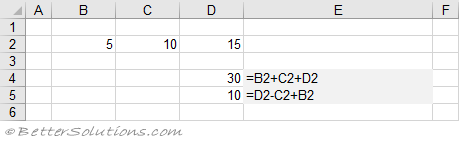### R1C1 Notation

This notation is also known as Relative Notation, not to be confused with Relative References.
This is an alternative way for creating (and displaying) cell references to other cells.
R1C1 cell references are displayed using Row and Column offset values.
Displaying your formulas this way often makes the relationships between the cells easier to understand.
This shows the same formulas but this time displayed in R1C1 notation.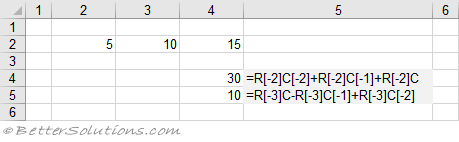The numbers inside the square brackets show how many rows or columns the cell is relative to itself.
Negative row numbers mean that the referenced cell is above the cell containing the formula.
Negative column numbers mean that the referenced cell is to the left of the cell containing the formula.

### Switching to R1C1 Notation

You can switch your cell references to the R1C1 notation from the Excel Options dialog box.
Select the Formulas tab and scroll down to the "Working with formulas" heading.
Select the "R1C1 reference style" checkbox.
Changing to R1C1 notation will not change the formulas. It will only change the view.
Changing this option will change the view of all the formulas in the active workbook.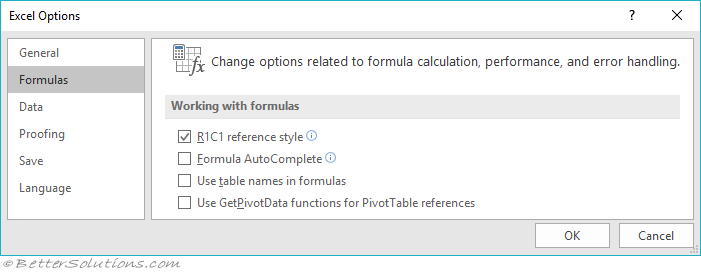### R1C1 Notation - Relative References

There are two different types of R1C1 Notation.
You can have relative references or absolute references.
Relative References ( R[-1]C[-1] ) include square brackets around the numbers.
This type is the default and they include square brackets around the numbers.
The two formulas below refer to different cells but have the same relative R1C1 notation.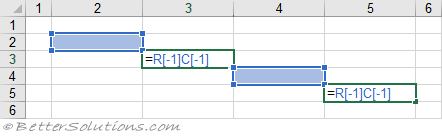### R1C1 Notation - Absolute References

Absolute References ( R2C2 ) do not include square brackets around the numbers.
The two formulas below refer to the same cell and have the same absolute R1C1 notation.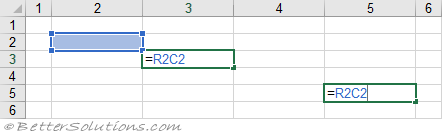### Comparing Notations

 In Cell A1 Formula R1C1 Formula A1 =B2 =RC A2 =B2+1 =RC+1 A3 =A2+1 =R[-1]C+1 B4 =\$A\$3+1 =R3C1+1 C5 =B\$4+1 =R4C[-1]+1 D6 =\$C5+1 =R[-1]C3+1 A6 =SUM(A1:A5) =SUM(R[-5]C:R[-1]C)

Although this is an older style of referencing cells it can be very useful for checking and finding any erroneous formulas.
This notation can be useful when you are more interested in the relative position of a cell rather than in its absolute position.

### Important

In R1C1 style, both columns and rows are labelled numerically.
References to cells and ranges do not have to be in the same worksheet as the formula.
While the R1C1 references are less compact the numerical references make it easier for Excel to calculate row and column offsets used in macros.
When you copy a formula, every copied formula will have exactly the same R1C1 notation.